# Class 12 Physics - Atoms

4128

Physics Modern Physics Level: Misc Level

Hydrogen atoms are usually found in the ground state, that is, the electron is in the n= 1 level . what wavelength light striking the atom will excite it to the n= 2 level?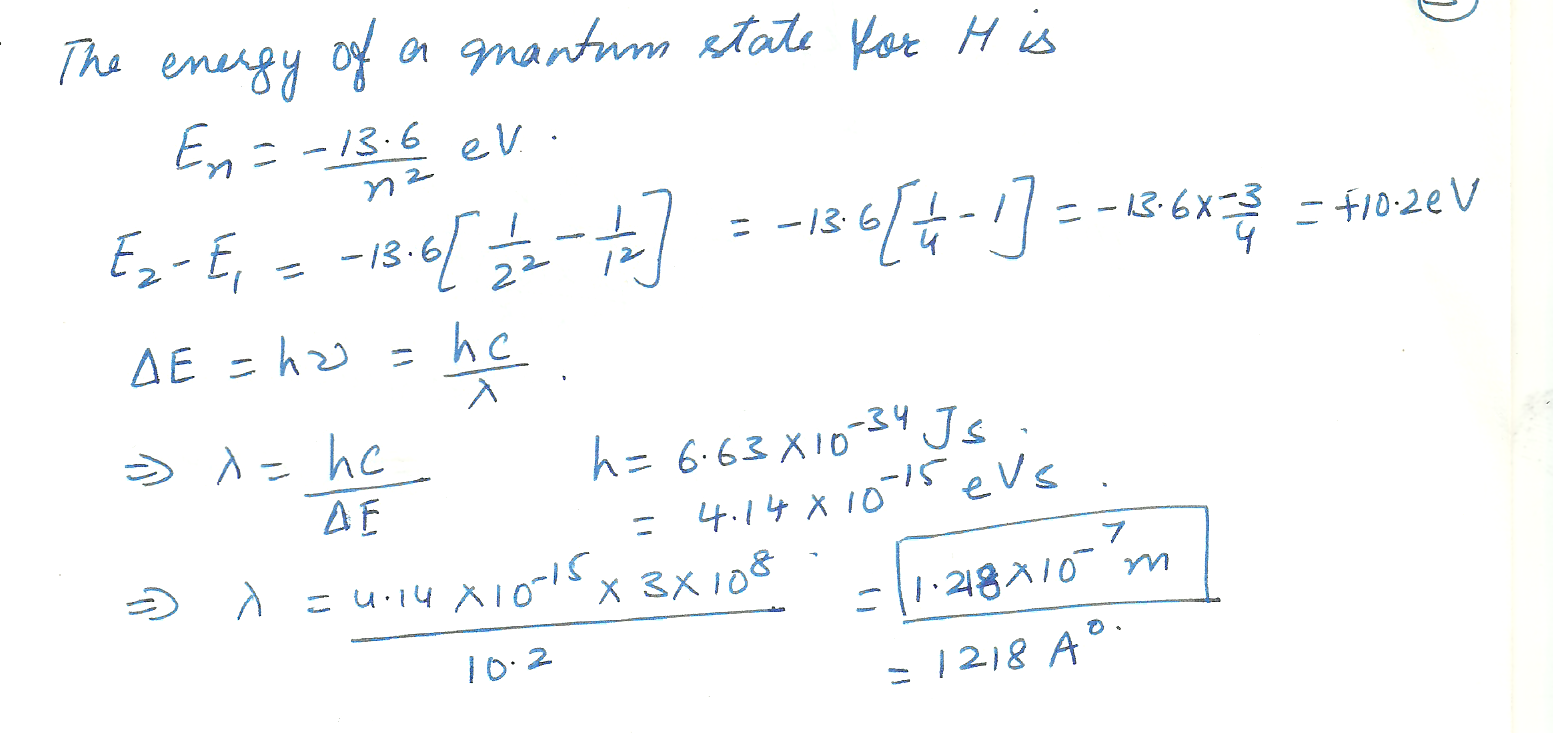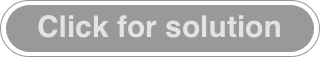3534

Physics Modern Physics Level: University

Atom
Find the energy for a hydrogen atom in the stationary state n=4.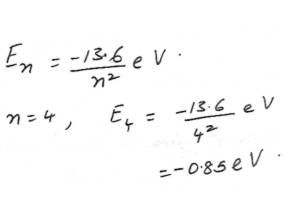2471

Physics Modern Physics Level: High School

Bohrâ€™s Atomic Model

Hydrogen emits a photon with wavelength lamda = 435 nm . Which transition between energy levels does this correspond to ?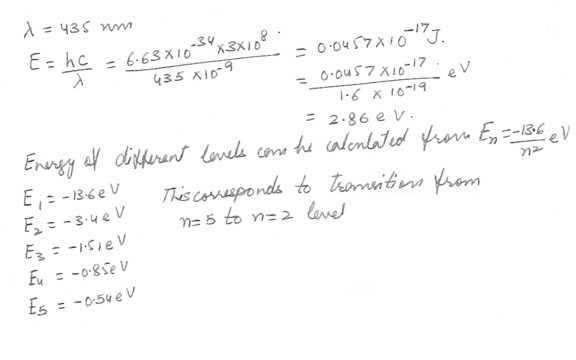2470

Physics Modern Physics Level: High School

Bohrâ€™s Atomic Model

What is the energy difference between an electron in n = 3 state and n = 5 state in hydrogen ? If the electron makes a transition from n = 5 to n = 3 this energy difference is carried away by a single photon . Calculate the wavelength expressed in nm , and the frequency of this emitted photon . In what part of the electromagnetic spectrum does it lie ?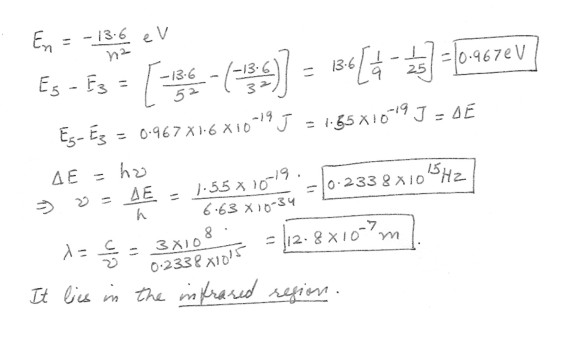2453

Physics Modern Physics Level: High School

A hypothetical atom has four energy states as shown in the figure at â€œclick here" Which of the following photon energies Ev could not be found in the emission spectra of this atom after it has been excited to the n = 4 state ?

1) Ev = 5 eV

2) Ev = 4 eV

3) Ev = 1 eV

4) Ev = 3 eV

5) Ev = 2 eV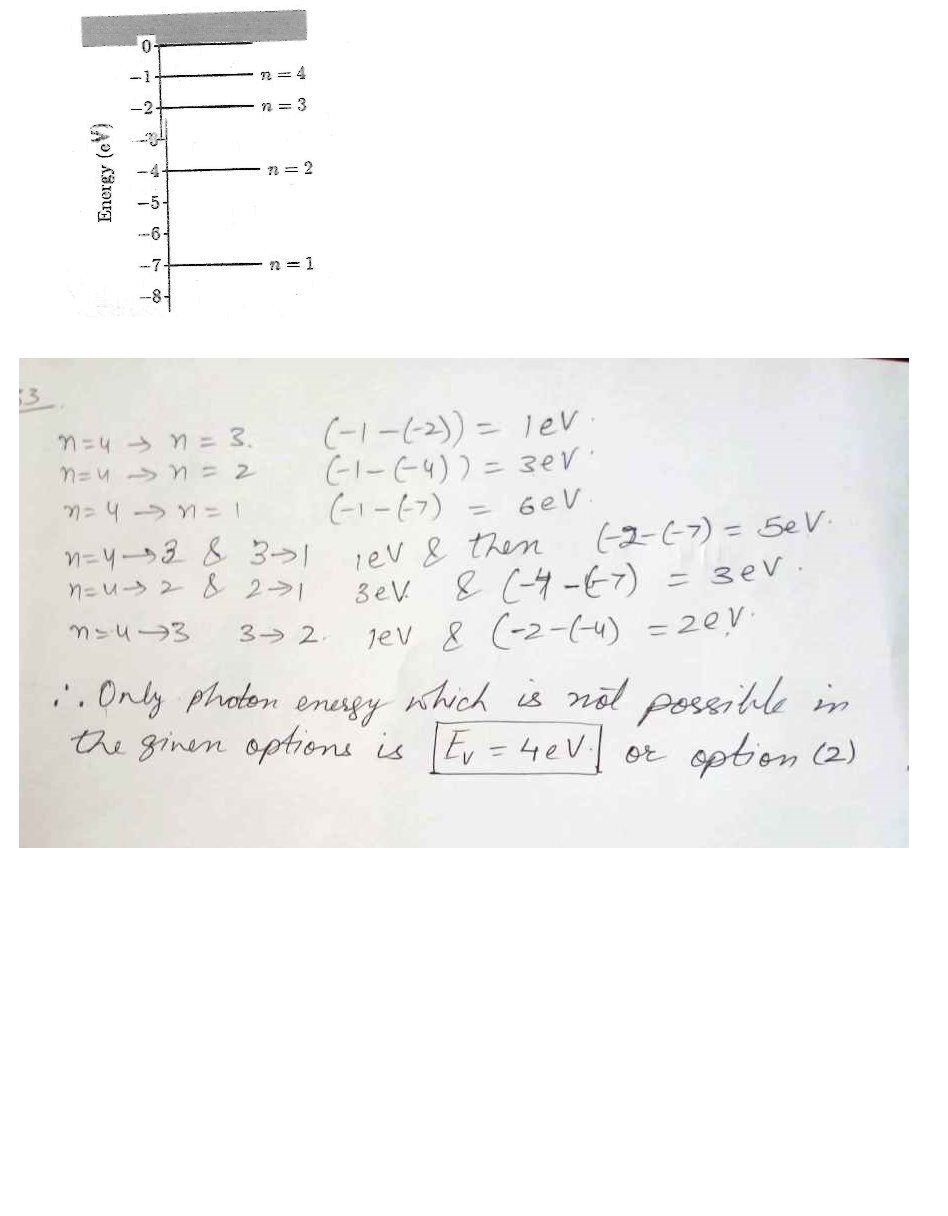2446

Physics Modern Physics Level: High School

Bohrâ€™s Atomic Model

The electron of a hydrogen atom is initially at the orbit n = 4 , If this electron first goes to the orbit n = 3 and then to the orbit n = 2 and then the orbit n =1 , how many photons are emitted during these transitions ? How many photons are emitted if the electron directly falls from the orbit n = 4 to the orbit n = 1 ?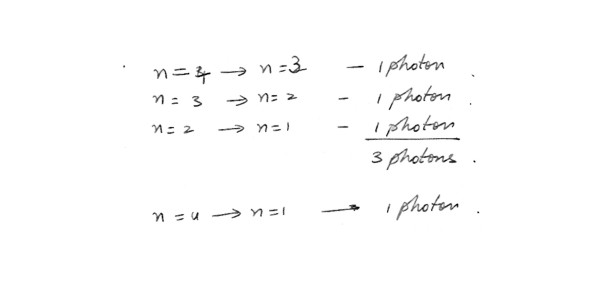2435

Physics Modern Physics Level: High School

Bohr's Atomic Model

A hydrogen atom is in the fifth excited state when it emits a photon with a wavelength of 1090 nm . Note than n = 2 is the first excited state . Calculate the energy of the atom in the final state .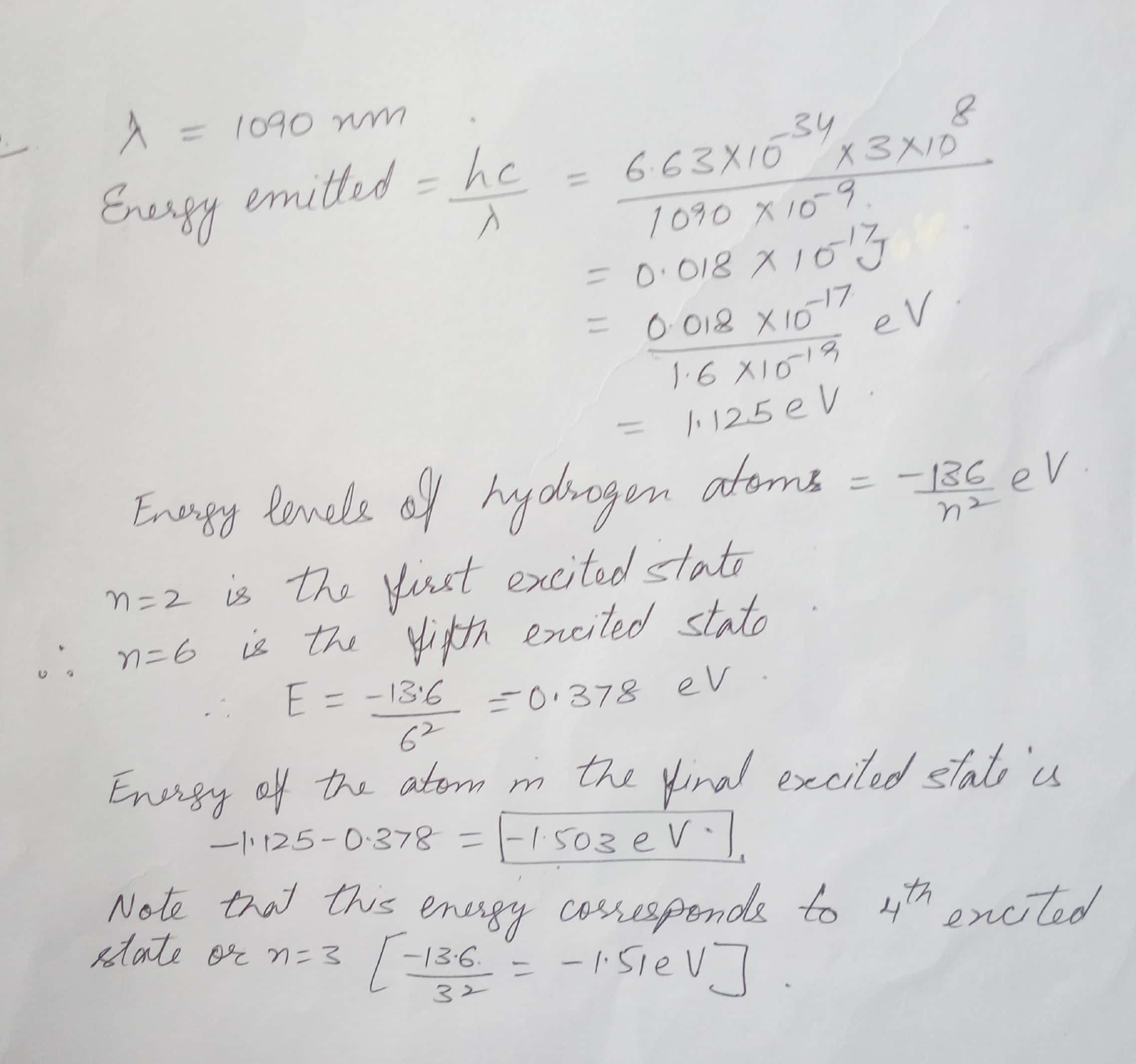2104

Physics Modern Physics Level: High School

Atom

The highest energy level in an atom is its

a) ionization level

b) emission level

c) absorption level

d) photon level

e) ground state

2102

Physics Modern Physics Level: High School

Atom

An excited atom gains energy by ?

a) emitting a photon

b) absorbing a photon

c) undergoing a photoelectric effect

d) increasing its de Broglie wavelength

e) the uncertainty principle

Displaying 1-9 of 9 results.